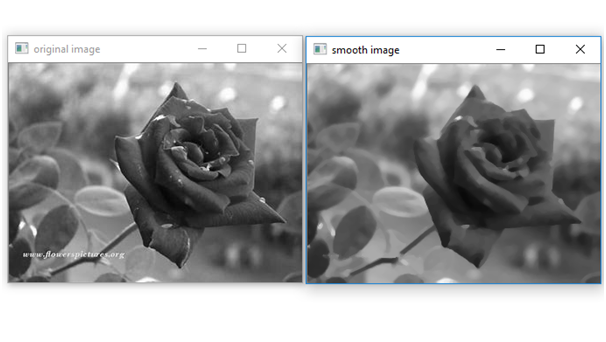# Smoothen a grayscale image by performing blurring operation using user defined median blur filter

In this article, we will see how to make user defined medium blur filter of required size and using this perform blurring operation on the image?
Submitted by Ankit Rai, on May 18, 2019

Image Blurring refers to making the image less clear or distinct. The Median Filter often used to remove noise from an image or signal. Median filtering is very widely used in digital image processing because, under certain conditions, it preserves edges while removing noise.

In this program, we will be using two functions of OpenCV-python (cv2) module.. let's see their syntax and descriptions first:

It takes an absolute path/relative path of your image file as an argument and returns its corresponding image matrix.

If flag value is:

• 1: Loads a color image.
• 0: Loads image in grayscale mode.
• -1: Loads image as such including alpha channel.

If the flag value is not given then show the original image, which path is given.

2) imshow():
It takes window name and image matrix as an argument in order to display an image in a display window with a specified window name.

Also In this program, we will be using one function of numpy module.

median(): It takes array and returns the median of the array.

Also, in this program we are using the concept of array slicing

Let, A is 1-d array:
A[start:stop:step]

1. start: Starting number of the sequence.
2. stop: Generate numbers up to, but not including this number.
3. step: Difference between each number in the sequence.

Example:

```    A = [1,2,3,4,5,6,7,8,9,10]
print(A[ 1: 5])

Output:
[2,3,4,5]
```

### Python program for smoothen a grayscale image

```# import cv2 module
import cv2

# import numpy module as np
import numpy as np

# Define a function for performing
# Median Blur on images
def MedianBlur(img,size) :
Ic = img

# run a loop from half of the size + 1 to  upto
# number of rows present in the image
for i in range(size//2 + 1, Ic.shape) :

# run a loop  from half of the size + 1 upto
# number of columns present in the image
for j in range(size//2 +1, Ic.shape) :

# Take a sub-matrix of specifed order form Ic image matrix
N = Ic[i-size//2 : i+ size//2 + 1, j - size//2: j+ size//2 + 1]

# find out median of submatrix
med = np.median(N)

# assing that medium value to the specified pixel coordinates
img[i, j] = med

# return blur image
return img

# Driver code
if __name__ == "__main__" :

# we have to  pass the path of the image
# and tha value of flag which is optional

# displaying the gray scale image
cv2.imshow('original image',img)

# order of the submatrix
order = 5

# MedianBlur function calling
img = MedianBlur(img,order)

# displaying the smoothen image
cv2.imshow("smooth image",img)
```

OutputPreparation